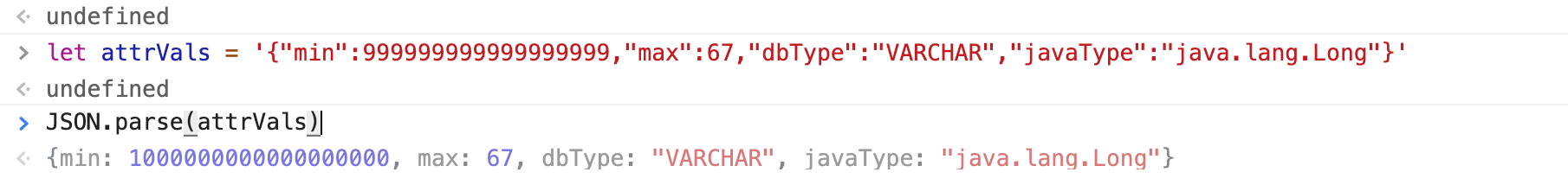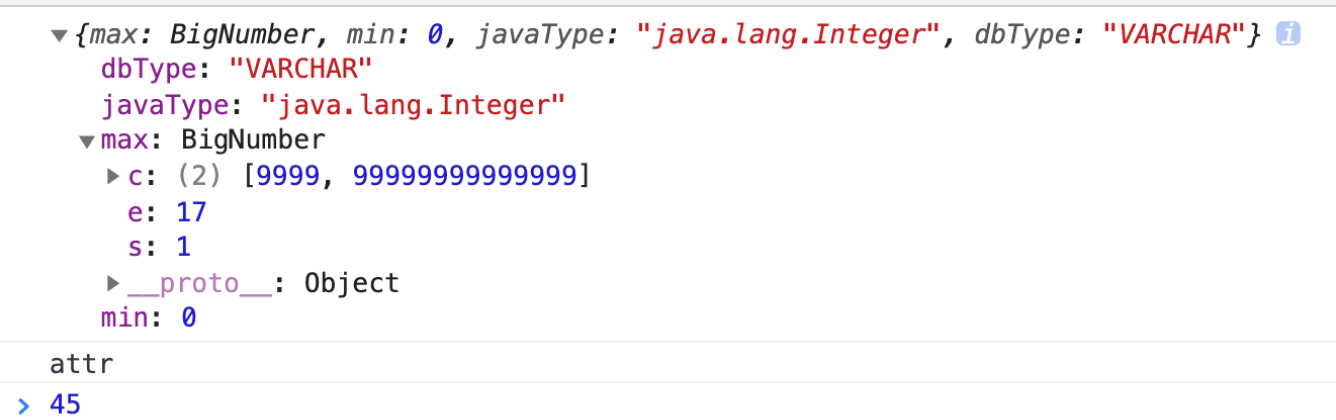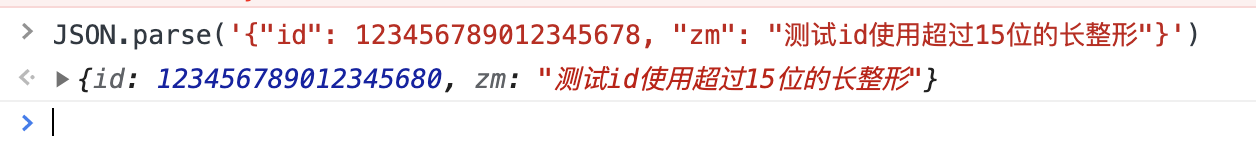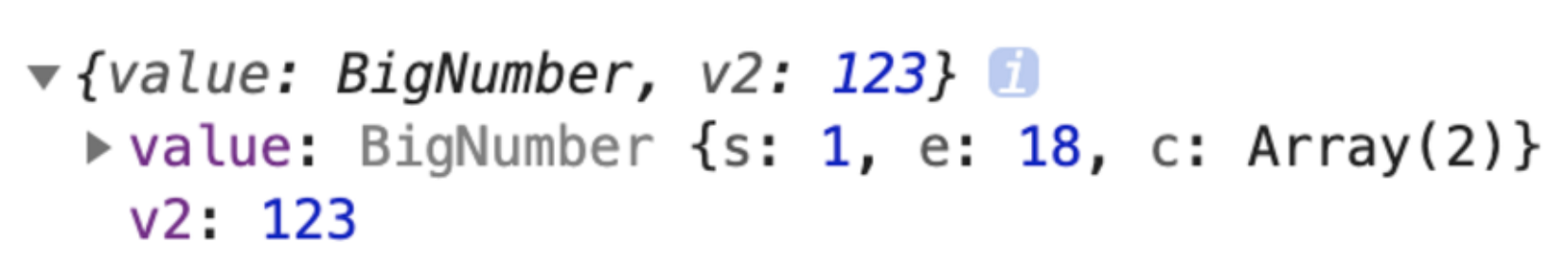# 两个大整数的比较，包括超过16位精度的数字（json-bigint的使用）

JS整数的精度是Math.pow(2,53),大于 9007199254740992 (16位数)的可能会丢失精度。所以对于大数字加减和比较，可以将数字拆分成多个15位数字，进行加减或比较。// json串里面的长整型使用bigInt转换成字符串
import JSONbig from 'json-bigint'
const result = JSONbig.parse(this.customData.attrVals)
max: result.max.toString(),// 每一段最多15位  如果有2段第一段从0 开始，第二段从str.length 开始
const getMidNum = (str:string, start:number, len:number) => {
if (start + len > 0) {
return +str.substr(start < 0 ? 0 : start, start < 0 ? start + len : len)
} else {
return 0
}
}

/**
* 比较两个大整数的大小，返回－1，0，1  a<b返回-1
* @param {String} a
* @param {String} b
* @returns {number}
*/
const bigNumCompare = (a:string, b:string): number => {
let back = 0
// 取最大值分15份，向上取整
let max = Math.ceil(Math.max(a.length, b.length) / 15)
// 分成多少段,从左边开始
for (let i = max; i > 0; i--) {
let num1 = getMidNum(a, a.length - i * 15, 15)
let num2 = getMidNum(b, b.length - i * 15, 15)
// 15位数字相减
let cur = num1 - num2
if (cur < 0) {
back = -1
break
} else if (cur > 0) {
back = 1
break
}
}
return back
}

export { bigNumCompare }


### json-bigint 的使用

JSON.parse() 把后端返回的数据转为 JavaScript 对象，一旦里面包含长整形超过16位，转换就会失精度

json-bigint 会把超出 JS 安全整数范围的数字转为一个 BigNumber 类型的对象，对象数据是它内部的一个算法处理之后的，我们要做的就是在使用的时候转为字符串来使用let zm = JSONbig.parse('{"id": 123456789012345678, "zm": "测试id使用超过15位的长整形"}')
zm.id.toString() //就可以取出大整数了

posted @ 2021-07-08 19:57  whkl梅  阅读(353)  评论(1编辑  收藏  举报